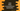# Java Math incrementExact explanation with example## Java Math incrementExact :

incrementExact is used to increment the value of an integer or double. This method is defined for both integer and doubles. Following are the definitions of this method :

``````public static int incrementExact(int value)

public static long incrementExact(long value)``````

This method is static. So, we can call it directly without creating an object.

The argument is the value we want to increment. This value is incremented by one and returns by these methods. If the result overflows an integer or long, it will throw one ArithmeticException.

### Example with integers :

``````public class Main {
public static void main(String[] args) {
System.out.println(Math.incrementExact(0));
System.out.println(Math.incrementExact(10));
System.out.println(Math.incrementExact(-30));
}
}``````

It will print the below outputs :

``````1
11
-29``````

### Example of ArithmeticException :

``````public class Main {
public static void main(String[] args) {
System.out.println(Math.incrementExact(Integer.MAX_VALUE));
}
}``````

This will throw one ArithmeticException as the result will exceed the maximum value of Integer.

### Example with long :

Similar to integers, we can use this method with long values like below :

``````public class Main {
public static void main(String[] args) {
System.out.println(Math.incrementExact(1000000000099888888L));
System.out.println(Math.incrementExact(999992823333333929L));
System.out.println(Math.incrementExact(-3099999999999999999L));
}
}``````

This will print the below output :

``````1000000000099888889
999992823333333930
-3099999999999999998``````

### Example of ArithmeticException with long :

Similar to integers, it will also throw one ArithmeticException if the result exceeds the maximum value of long.

``````public class Main {
public static void main(String[] args) {
System.out.println(Math.incrementExact(Long.MAX_VALUE));
}
}``````